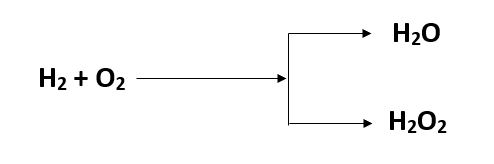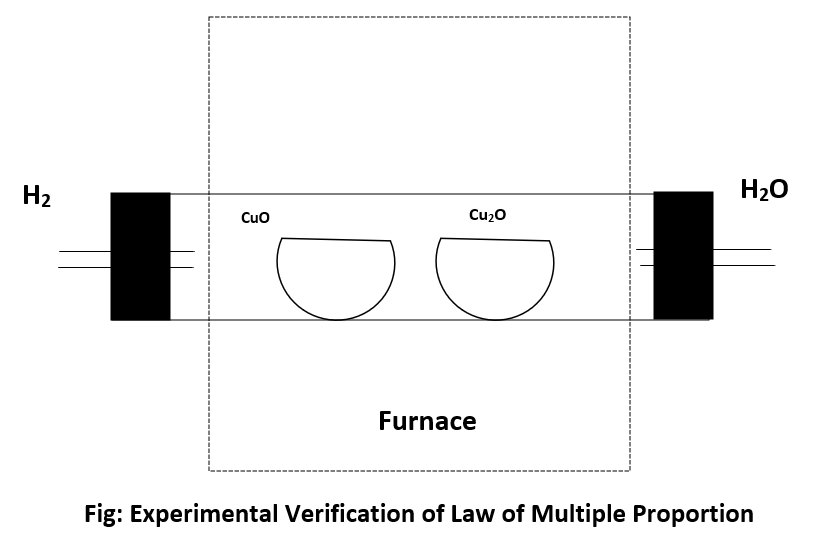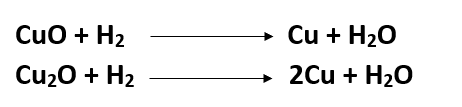Law of Multiple Proportion↪ This law states that, "When two elements combined to each other to give two or more products then the ratio of mass of one element in this compounds which combine with fixed mass (same mass) of another element bear simple whole number ratio."

For example: Hydrogen combines with oxygen to give H2O and H2O2 then the ratio of mass of O2 in H2O and H2O2 which combine with 1gm of H is 1:2 which is simple whole number ratio.Calculation:
In H2O, 2gm of H combined with 16gm of O2
In H2O2, 2gm of H combined with 32gm of O2

⇒Ratio of mass of oxygen in H2O and H2O2 = 16:32 = 1:2 which is simple whole number ratio.

⁕ ﻿Experimental Verification of Law of Multiple proportion.First of all, known weight of cupric oxide (CuO) and cuprous oxide (Cu2O) are taken in two different porcelain basin and are completely reduced with hydrogen in a sealed tube. The weight of oxygen present in each oxide can be calculated as:Wt. of O2 = Wt. of oxide - wt. of Cu ( in CuO or Cu2O)

Then the ratio of mass of copper present in CuO and Cu2O is taken where the mass of oxygen in both the compounds (oxides) is kept constant.

If simple whole number ratio of the mass of the copper is obtained then the law is verified.